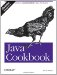# Introduction

Numbers are basic to just about any computation. They're used for array indexes, temperatures, salaries, ratings, and an infinite variety of things. Yet they're not as simple as they seem. With floating-point numbers, how accurate is accurate? With random numbers, how random is random? With strings that should contain a number, what actually constitutes a number?

Java has several built-in types that can be used to represent numbers, summarized in Table 5-1. Note that unlike languages such as C or Perl, which don't specify the size or precision of numeric types, Java with its goal of portability specifies these exactly and states that they are the same on all platforms.

##### Table 5-1. Numeric types

Built-in type

Object wrapper

Size of built-in (bits)

Contents

byte

Byte

8

Signed integer

short

Short

16

Signed integer

int

Integer

32

Signed integer

long

Long

64

Signed integer

float

Float

32

IEEE-754 floating point

double

Double

64

IEEE-754 floating point

char

Character

16

Unsigned Unicode character

As you can see, Java provides a numeric type for just about any purpose. There are four sizes of signed integers for representing various sizes of whole numbers. There are two sizes of floating-point numbers to approximate real numbers. There is also a type specifically designed to represent and allow operations on Unicode characters.

When you read a string from user input or a text file, you need to convert it to the appropriate type. The object wrapper classes in the second column have several functions, but one of the most important is to provide this basic conversion functionality replacing the C programmer's atoi /atof family of functions and the numeric arguments to scanf .

Going the other way, you can convert any number (indeed, anything at all in Java) to a string just by using string concatenation. If you want a little bit of control over numeric formatting, Recipe 5.8 shows you how to use some of the object wrappers' conversion routines. And if you want full control, it also shows the use of NumberFormat and its related classes to provide full control of formatting.

As the name object wrapper implies, these classes are also used to "wrap" a number in a Java object, as many parts of the standard API are defined in terms of objects. Later on, Recipe 10.16 shows using an Integer object to save an int's value to a file using object serialization, and retrieving the value later.

But I haven't yet mentioned the issues of floating point. Real numbers, you may recall, are numbers with a fractional part. There is an infinity of possible real numbers. A floating-point number what a computer uses to approximate a real number is not the same as a real number. The number of floating-point numbers is finite, with only 2^32 different bit patterns for floats, and 2^64 for doubles. Thus, most real values have only an approximate correspondence to floating point. The result of printing the real number 0.3 works correctly, as in:

`// RealValues.java System.out.println("The real value 0.3 is " + 0.3);`

results in this printout:

`The real value 0.3 is 0.3`

But the difference between a real value and its floating-point approximation can accumulate if the value is used in a computation; this is often called a rounding error . Continuing the previous example, the real 0.3 multiplied by 3 yields:

`The real 0.3 times 3 is 0.89999999999999991`

Surprised? More surprising is this: you'll get the same output on any conforming Java implementation. I ran it on machines as disparate as a Pentium with OpenBSD, a Pentium with Windows and Sun's JDK, and on Mac OS X with JDK 1.4.1. Always the same answer.

And what about random numbers? How random are they? You have probably heard the expression "pseudo-random numbers." All conventional random number generators, whether written in Fortran, C, or Java, generate pseudo-random numbers. That is, they're not truly random! True randomness comes only from specially built hardware: an analog source of Brownian noise connected to an analog-to-digital converter, for example. This is not your average PC! However, pseudo-random number generators (PRNG for short) are good enough for most purposes, so we use them. Java provides one random generator in the base library java.lang.Math , and several others; we'll examine these in Recipe 5.13.

 For a low-cost source of randomness, check out http://www.lavarand.org. These folks use digitized video of 1970s "lava lamps" to provide "hardware-based" randomness. Fun!

The class java.lang.Math contains an entire "math library" in one class, including trigonometry, conversions (including degrees to radians and back), rounding, truncating, square root, minimum, and maximum. It's all there. Check the Javadoc for java.lang.Math.

The package java.Math contains support for " big numbers" those larger than the normal built-in long integers, for example. See Recipe Recipe 5.19.

Java works hard to ensure that your programs are reliable. The usual ways you'd notice this are in the common requirement to catch potential exceptions all through the Java API and in the need to "cast" or convert when storing a value that might or might not fit into the variable you're trying to store it in. I'll show examples of these.

Overall, Java's handling of numeric data fits well with the ideals of portability, reliability, and ease of programming.

The Java Language Specification. The Javadoc page for java.lang.Math.Java Cookbook, Second Edition
ISBN: 0596007019
EAN: 2147483647
Year: 2003
Pages: 409
Authors: Ian F Darwin

Similar book on Amazon